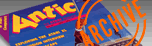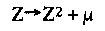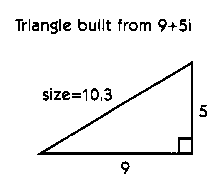`ANTIC VOL. 4, NO. 12 / APRIL 1986`

# FRACTALS FOR YOUR ATARI

## MATHEMATICS IN PICTURE FORM

BY CHARLES JACKSON ANTIC PROGRAM EDITOR

Everybody's talking about fractals-what a breakthrough these complicated "non-Euclidean" mathematical shapes are for creating realistic computer graphics.
But few people really understand what fractals are, or how they're used to simulate natural phenomena in new computer games such as LucasFilm's Rescue on Fractalus and Koronis Rift.

WHY STUDY FRACTALS?
Fractal theory forms a primary link between mathematics and nature, a link that conventional mathematics had long been straining to achieve.
Coastlines are not curves, trees are not tubes, and clouds are not globes. But, all of these are fractal shapes which can be described and simulated with mathematical formulas.
Fractal theory is being used to study atmospheric turbulence, patterns of arteries and veins, and the distribution of stellar systems throughout the universe.
In the simplest of terms, fractals imitate nature.

WHAT IS A FRACTAL?
Fractals are shapes which are "infinitely squiggly."
Imagine a shape with an infinite perimeter (outer edge), but a finite area. You might draw a circle around such a shape in a moment, but you'd need an eternity to trace it precisely
The coastline of Britain is a popular example of a fractal. In the following mental exercise, our task will be to find the exact length of this coastline.
This is not as simple as it sounds. Coastlines are usually quite irregular, and cannot be represented with smooth curves. Every inlet, bay and peninsula contributes to the total length of a coastline.
We can estimate the length of a coastline with a satellite picture of the island. Unfortunately, a photograph taken from that altitude would not show all of the bays and peninsulas which would contribute to the length of the coastline.
So let's come a little closer.
If we drove a car around the coastline of Britain, keeping our left wheels in the water and our right wheels on the beach, our total mileage would be a better estimate of its length. But it would still be an estimate. We'd still miss the countless tiny bumps and irregularities too small to drive around accurately.
We'd run into the same problem if we walked around the coastline, crawled around the coastline with a ruler, or measured every bit of the coastline through a microscope. No matter how closely you examined it, there would always be wrinkles and bulges beyond the range of your instruments, and these wrinkles and bulges would contribute to the coastline's length.
In the real world, we can imagine "zooming in" on a coastline until we're looking at molecules and atoms. In the realm of mathematics, we deal with numbers, and our imaginary "zoom lens" is no longer limited by the size of atomic particles. We can "zoom-in" on a mathematical coastline infinitely. The shape defined by such a coastline is fractal.

EXAMPLE:
Consider points A and B on this mathematical coastline. From a satellite picture taken at an altitude of 200 miles, we estimate that there are 10 miles of coastline between the two points. A satellite picture taken at 100 miles reveals many smaller bays and peninsulas too small to be seen at higher levels. From this new information, we now estimate the length of the coastline between A and B to be 15 miles.

HAUSDORFF DIMENSION
Mathematicians put both estimates into a complex formula which yields a number called the Hausdorff Besicovitch dimension-D. The Hausdorff dimension acts like a ratio of the new estimate to the old estimate. (In the previous example, D is approximately equal to 1.176.)
In other words, if we are zooming in on a coastline at a constant speed, the Hausdorff dimension is proportional to the rate at which our coastline estimates grow. If we discover only a handful of new bays and peninsulas each time we zoom, D will be slightly greater than one.
On the other hand, if we discover a great many bays and peninsulas with each zoom, D will be slightly less than two.
As D approaches two, however, the coastline become so irregular that our bays begin to close into lakes, and our peninsulas begin to split off into islands. Since lakes and islands are not part of a coastline, D must be greater than one, but less than two.
The topological dimension, DT, refers to the definition of "dimension" we learn in basic geometry, such as two-dimensional shapes and three-dimensional shapes. Circles, for example, have a topological dimension of two, while spheres have a topological dimension of three.
The Hausdorff dimension of all simple geometric shapes (including circles, polygons and ellipses) is always equal to the topological dimension.
A fractal is defined as a shape whose Hausdorff dimension is greater that its topological dimension.
In other words, fractals have fractional Hausdorff dimensions. Although Benolt Mandelbrot created the term fractal from the Latin fractus, meaning "fragmented" or "irregular," many authors have also used the term as a mnemonic device for "fractional Hausdorff dimension."

JULIA FRACTAL CURVES
Perhaps the most celebrated fractal shapes are the Julia Fractal Curves, nicknamed the Mandelbrot Set. The fractal images in this issue are examples of such curves.
The curves are created through an iterative process published in 1906 by French mathemeticians Gaston Julia and Pierre Fatou.
An iterative process is a task done over and over again until one or more conditions are met. A FOR-NEXT loop is a good example of an iterative process.
This iterative process we're concerned with, called self-squaring, is based on the formula:Here, Z and u are complex numbers. Z refers to a particular point in the complex plane, and u is a complex constant. (See the accompanying story for more information about complex numbers).
Our iteration has several steps: For every point Z, we will:

1. Set a counter equal to zero.
2. Multiply Z by itself, and add u.
3. Set Z equal to this new value.
4. Calculate the size of Z.
5. Increase the counter by one.
6. If the size of Z is greater than or equal to 2, jump to step 9.
7. If the counter is greater than 100, jump to step 9.
8. Goto step 2.
9. Stop iterating and remember the value of the counter.

Once we reach step nine, if the value of the counter is greater than 100, our point lies within the Julia curve. Such points are conventionally colored black.
Other counter values will produce other colors. For example, points which yield counter values between 0 and ten may be colored red, values between 11 and 25 might be colored blue, and so on.
By performing this iteration on every point on the computer screen, we can create our own Julia curves. We can also vary our starting coordinates and the complex constant, u, to create an infinite variety of fractal shapes.
The programs in this issue will help you create your own Julia curves. The 3-D fractal program, written for the 520ST, creates striking three-dimensional fractal images which closely resemble rugged mountain ranges, colorful valleys and winding rivers.
The Fractal Zoom program, written for 8-bit Atari computers, creates fractal shapes in a variety of graphics modes, and then lets you continually "zoom-in" on any part of them.

## COMPLEX NUMBERS EXPLAINED

Meet the square root of -1
Engineers and mathematicians use complex numbers to deal with many involved functions and algorithms. A complex number, such as 4+ 6i, is a number made of two parts-a real part (4) and an imaginary part (6i).
A real number is a common, ordinary number, such as 12, -.003 and 22/7. We count with real numbers, do our taxes with them and use them for numbering magazine pages.
An imaginary number is a real number multiplied by i, where i is the square root of -1. In the physical world, negative numbers do not have square roots-hence the name "imaginary." Although it's difficult to visualize i, it is frequently used in many equations, including the ones which generate our self-squared Julia fractal curves.
These curves are mapped onto the complex coordinate plane or grid. A complex coordinate plane looks like a piece of graph paper. One coordinate represents the real part of a complex number, and the other coordinate represents the imaginary part. For example, the complex number 9 + 5i would be plotted nine places to the right, and five places up. This is analagous to plotting the point (9,5) on an (x-y) grid. The complex number 0 + 0i would be plotted at the (0,0) point of this graph.

COMPLEX NUMBER SIZE
The size of a complex number refers to its distance from 0 + 0i. We can visualize this by imagining a right triangle in which the length of one leg is equal to the real part of a complex number, and the length of the other leg is equal to the length of the imaginary part. The size of this complex number would be equal to the length of the triangle's hypotenuse.We can use the Pythagorean Theorem to determine this value. In the above example, we used the complex number 9 + 5i. The size of this number is equal to: SQR(92+ 52) or approximately 10.3.-Charles Jackson